GeeksforGeeks App
Open AppBrowser
Continue

# How to use Summary Function in R?

In this article, we will discuss the Summary Function in R Programming Language.

Summary function is used to return the following from the given data.

• Min: The minimum value in the given data
• 1st Qu: The value of the 1st quartile (25th percentile) in the given data
• Median: The median value in the given data
• 3rd Qu: The value of the 3rd quartile (75th percentile) in the given data
• Max: The maximum value in the given data

Syntax:

`summary(data)`

Where, data can be a vector, dataframe, etc.

### Example 1: Using summary() with Vector

Here we are going to create a vector with some elements and get the summary statistics.

## R

 `# create a vector with 10 elements``data = ``c``(1: 5, 56, 43, 56, 78, 51)` `# display``print``(data)` `# get summary``print``(``summary``(data))`

Output: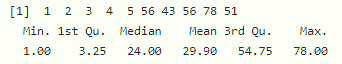### Example 2: Using summary() with DataFrame

Here we are going to get the summary of all columns in the dataframe.

## R

 `# create a dataframe with 3 columns``data = ``data.frame``(col1=``c``(1: 5, 56, 43, 56, 78, 51),``                  ``col2=``c``(100: 104, 56, 43, 56, 78, 51),``                  ``col3=``c``(1: 5, 34, 56, 78, 76, 79))` `# display``print``(data)` `# get summary``print``(``summary``(data))`

Output: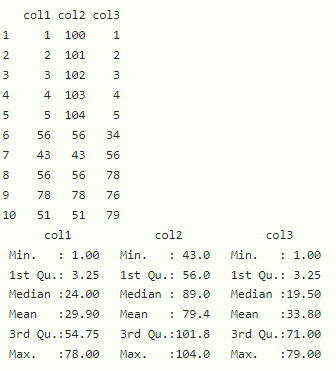### Example 3: Using summary() with Specific DataFrame Columns

Here we can get summary of particular columns of the dataframe.

Syntax:

`summary(dataframe)`

## R

 `# create a dataframe with 3 columns``data = ``data.frame``(col1=``c``(1: 5, 56, 43, 56, 78, 51),``                  ``col2=``c``(100: 104, 56, 43, 56, 78, 51),``                  ``col3=``c``(1: 5, 34, 56, 78, 76, 79))` `# display``print``(data)` `# get summary of column 1 and column 3``print``(``summary``(data[``c``(``'col1'``, ``'col3'``)]))`

Output: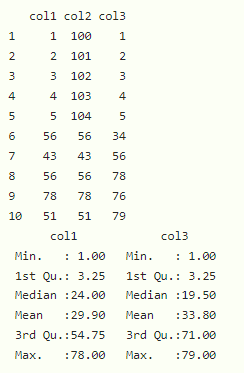### Example 4: Using summary() with Regression Model

Here we can also calculate summary() for linear regression model. We can create an linear regression model for dataframe columns using lm() function.

Syntax:

`summary(lm(column1~column2, dataframe))`

## R

 `# create a dataframe with 3 columns``data = ``data.frame``(col1=``c``(1: 5, 56, 43, 56, 78, 51),``                  ``col2=``c``(100: 104, 56, 43, 56, 78, 51))` `# create the model for regression with 2 columns``reg = ``lm``(col1~col2, data)` `# get summary of the model``summary``(reg)`

Output: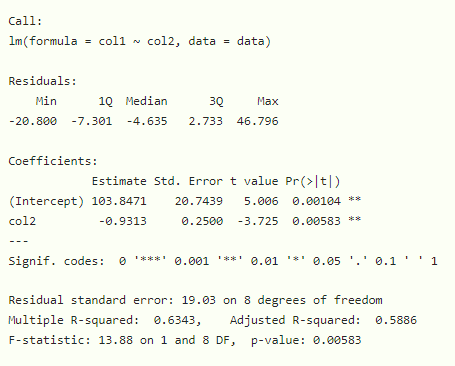### Example 5: Using summary() with ANOVA Model

Here aov() is used to create anova model which stands for analysis of variance.

Syntax:

`summary(aov(col1 ~ col2, data))`

Example:

## R

 `# create a dataframe with 3 columns``data = ``data.frame``(col1=``c``(1: 5, 56, 43, 56, 78, 51),``                  ``col2=``c``(100: 104, 56, 43, 56, 78, 51))` `# create the model for anova model with 2 columns``reg = ``aov``(col1 ~ col2, data)` `# get summary of the model``summary``(reg)`

Output: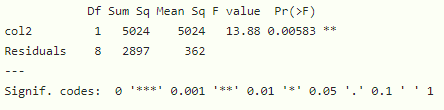My Personal Notes arrow_drop_up